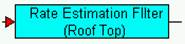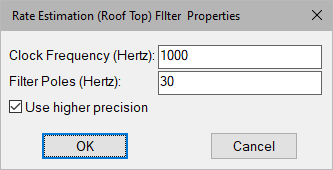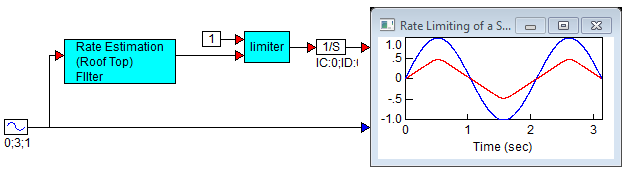### Rate Estimation (Rooftop) FilterCategory: Other Tools > Filters

The Rate Estimation (Rooftop) Filter block provides a band limited derivative of a signal by band-pass filtering. This method of obtaining the derivative is optimal in a sense that it maximizes derivative bandwidth as a compromise to upper band noise attenuation. The rooftop filter is a 2-pole band pass filter in which the poles at p rad/sec are equal. If the filter is scaled by p2, the low frequency band of the filter well below the filter poles corresponds to the response of a pure derivative with gain equal to ω and a phase shift of plus 90o. As the frequency nears p rad/sec, phase shift becomes less than 90 o and the derivative response is diminished. Frequency content above p rad/sec is attenuated at –20 dB/decade. A general rule is to select the filter pole 30 times larger than the highest frequency at which derivative response is to be applied. Pole selection provides a trade-off between derivative bandwidth and higher frequency noise attenuation.Clock Frequency: Defines the processor rate for rate estimation in hertz.

Filter Pole: Defines the center frequency of the rooftop filter in hertz. Select a frequency 30 times larger than the highest frequency for estimation of derivative.

#### Example

Diagram name: Rate Estimation Roof Top Filter

Location: Examples > eMotor > Filters

The following example demonstrates how to use a rooftop filter to limit the rate on a signal. The rooftop filter is first used to determine the derivative (rad/sec) of the angular displacement input signal (rad). The rate is limited, and then converted back to radians by integration. The resulting signal shows limiting when the input signal exceeds the rate limit.